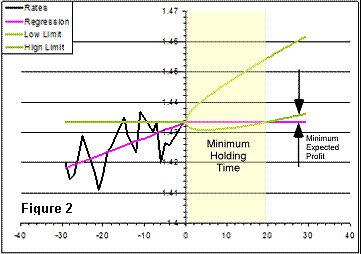# A Trend Tool

By Armando Rodriguez

This trend tool takes a date, a pair and a period and delivers a slope for the ask (quotes) regression and the minimum holding time. The period has following fixed choices: year, month, week, day and hour.

As the period increases, the number of samples to process also increases. In the average, there are about 50 quotes every minute for each pair, adding up to roughly 26 million in a year. This is way more than it is needed for a linear regression, the tool will keep the sample count below 100000. So, for hourly and daily periods, all the quotes will be processed; for weekly periods one of every five; for monthly,  one for every 21 and for yearly periods one every 262. The actual skipping factor is calculated as:

Skip = totalQuoteCount/100000

Only those quotes being issued in the seconds that are integer multiples of the skip will be processed.

For calculating the regression estimators, the following averages over the selected samples must be calculated

avgT       average of the times within the period

avgT2     average square time within the period

avgQ      average of the quotes

avgTQ   average of the products of the quote and its time

According to the linear regression formula, the slope S is:

S = (avgTQ - avgT*avgQ)/( avgT2 – (avgT)2)

The intercept  I :

I =  avgQ – slope*avgT

The equation for the regression line is:

Q(t) = S*t + I

The randomness about the regression line must now be estimated. This uncertainty (U) can be represented by the square root of the average of the squares of the residuals, which, assuming normality of the distribution, the standard deviation of the quotes from the perfect linear behavior. A residual is the difference between the actual quote and the regression line so:

U = Sn=1toN (Qn – (S*tn + I))2/N

Considering that the random part of the quotes motions is a Markov Chain, the next element in the sequence only depends on the present one, so any forecast must start from the last quote and the trend must show as:

q(t) = q(0) + S*t

From that moment on, still assuming normality, the probability of finding the quotes away from that last quote will follow a Gaussian function . This Gaussian broadens into the future with the square root of time. If the randomness stays the same, the standard deviation (u(t)) must reach the same value as U in the same period T (hour, day, week, etc.).

u(t) = (U/ÖT) *Ö t

The probability of getting a quote within the interval of +/- the standard deviation around the trend line is about 68%, that of getting one within twice the standard deviation is 95%.  Using this last interval of confidence, the upper limit (B(t)) or best case scenario for a buy as a function of time will be:

B(t) = q(t) +2* u(t)  = q(0) +  (S*t) + (2*U/ÖT) *Ö t

The lower limit of the 95% confidence interval (W(t)) or worst case scenario for a buy, will then be:

W(t) = q(t) - 2*u(t)  =  q(0) + (S*t) - (2*U/ÖT) *Ö tFor small values of t, W(t) will less than q(0), meaning that, for a buy, there’s a chance within the confidence interval for a loss. In other words, the time is too short for the trend to reveal over the randomness. There is a minimum time that a position must be held for the whole interval of confidence to go above the initial quote. Calling this the minimum holding time or MHT:

MHT = 4*U2/(T*S2)

A sell scenario would involve a negative trend and then the worst case would be the upper limit. A plot of the forecast consists of an inclined parabola ant the MHT would be the intercept with the last quote level.